#### Write short notes on:

###### i) Implications of business and financial risks

Business risk is the riskiness on a firm’s stock provided that the firm has used no debt capital. It is the risk inherent in the operation of the business. A firm’s business risk arises because of uncertainty associated with projections of return on invested capital. Return on investment varies due to the number of factors such as variability in demand, price of the product and cost of inputs, general economic condition, competition and so on. The degree of operating leverage (DOL) shows the extent of a firm’s business risk. Generally a firm with higher fixed operating costs has a higher degree of operating leverage. Firms with higher fixed operating costs must have larger sales to meet the fixed cost. At lower sales levels such firms are not able to meet the fixed operating costs. Such firms are exposed to higher levels of business risk. Thus the implication of business risk is that if the market is price sensitive, a firm with higher DOL can raise its profits by cutting the price. Conversely, a firm with higher DOL is unable to maximize sales, it may have to suffer substantial losses. This shows that the firm with higher DOL is exposed to a high degree of business risk.

Financial risk is the additional risk to the common stockholders due to the use of debt financing. Financial risk encompasses both the risk of possible insolvency and the added variability in ROE that is induced by the use of debt. Use of debt increases the proportion of fixed cost financing in its capital structure and it increases fixed cash outflows of the firm. As a result, the use of debt increases the probability of bankruptcy of the firm. Thus, the financial risk is the chance that the firm will be unable to cover its financial charges with cash earnings. One measure of the financial risk is the degree of financial leverage: A firm with higher fixed financing costs, such as interest and preferred stock dividend, has higher degree of financial leverage. The implication of using higher debt is that a firm with higher financial leverage can magnify its ROE with successful use of debt. Debt carries fixed interest and amount earned in excess of fixed interest is available to the common stockholders, hence their return is maximized. However, if the firm is not successful in using debt, interest expense remains a fixed financial burden. The use of excessive debt increases the financial risk to the common stockholders, because they may feel their position insecure with the increased debt.  financial leverage affects the return and financial risk for stockholders.

Factors affecting target capital structure

The target capital structure of a firm is affected by various factors as described below:

• Business risk: The level of business risk is determined by the use of fixed operating cost or operating leverage. The chance of business failure is higher for the firm with a higher level of business risk. Thus they tend to use lower debt.
• Cash flow stability: A firm with relatively stable cash flows finds no difficulties in meeting its fixed charge obligation. Thus such firms tend to use more debt.
• Firm size: The larger firms have easy access to the capital market as they have higher credit ratings for debt issues. Therefore they tend to use more debt capital than smaller firms.
• Sales growth: The firms with significant growth in sales have high market price per share. Thus they prefer to use more equity.
• Control and risk: Management’s attitude towards control and risk also affect capital structure decisions. If management wants to maintain control in the firm, they prefer to use more debt financing. Similarly, if the management of a firm is more risk seeker, they attempt to use more debt to take the advantage of financial leverage.
• Debt service capacity: Debt service capacity of the firm is indicated by interest coverage ratio. The firms with higher interest coverage ratio tend to use more debt.
• Asset structure: The maturity structure of assets to be financed also affects the capital structure. A firm with relatively higher longer-term assets and stable demand for products tends to use more long-term debt. On the other hand, a firm with relatively higher investment in receivables and inventory tends to use more short-term funds.
###### ii) Financial risk

A firm generally uses both debt and equity capital to finance its assets. The firm that uses debt capital in its capital structure is called a levered firm. Addition of debt capital to capital structure increases the chances of variability of return on capital. It means debt financing further increases the risk to the shareholders. Thus, financial risk is the additional risk to the common stockholders due to the use of debt financing. If a firm uses no debt there would be no financial risk. Financial risk encompasses both the risk of possible insolvency and the added variability in ROE that is induced by the use of debt. Use of debt increases the proportion of fixed cost financing in its capital structure and it increases fixed cash outflows of the firm. As a result, the use of debt increases the probability of bankruptcy of the firm. Thus, the financial risk is the chance that the firm will be unable to cover its financial charges with cash earnings:

One measure of financial risk is the degree of financial leverage. A firm with higher fixed financing costs, such as interest and preferred stock dividend, has a higher degree of financial leverage. As the firm employs more and more debt, its interest expenses and return on equity also increase correspondingly. It implies that, in any capital structure, interest expenses and return on equity increase with financial leverage. Higher the financial leverage, more the proportionate change in EPS and ROE for a given level of change in sales and EBIT. The implication of using higher debt is that a firm with higher financial leverage can magnify its ROE with successful use of debt. Debt carries fixed interest and amount earned in excess of fixed interest is available to the common stockholders, hence their return is maximized. However, if the firm is not successful in using debt, interest expense remains as the fixed financial burden. The use of excessive debt increases the financial risk to the common stockholders, because they may feel their position insecure with the increased debt. Thus, financial leverage affects the return and financial risk for stockholders.

## Numerical Problems

1) Northern manufactures gasoline additives. The materials cost is Rs. 1.15 per pint and the labor cost is Rs. 2.60.

a. What is the variable cost per unit?

b. Suppose northern incurs fixed costs of Rs. 320,000 during a year when total production is 280,000 pints. What are the total costs for the year?

c.  If the selling price is Rs. 5.30 per pint, and depreciation for the year is Rs. 130,000 per year, what is the accounting break-even point?

d. Does Northern break even on a cash basis?

SOLUTION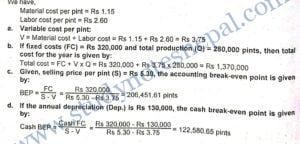2) A Project is projected to breakeven on an according basis in its third year. Sales for the year are projected at 12,000 units. Depreciation at that time will be Rs. 13,000. The price per unit less variable cost per unit is Rs. 15. What will be the fixed costs? If fixed cost is increased by 10 percent, what is its breakeven point in units?

SOLUTION

We have,

Break-even point (BEP) = 12,000 units (that is, third year’s sales)

Contribution margin per unit (S – V) = Rs 15

Annual depreciation (Dep.) = Rs 13,000

Calculation of fixed cost (FC):

We know,

BEP = FC /  S-V

12,000 = FC / Rs 15

FC = Rs 180,000

If cash fixed cost increased by 10 percent:

New FC = Rs 180,000+ 0.10 x Rs 180,000 = Rs 198,000

Hence,

BEP = New FC /  S-V =  Rs 198,000 / Rs 15 = 13,200 units

3) Yeti Carpet Mfg. Co. provides you the following information:

a. The company sold in two successive periods 1400 units of carpet of standard size and 1800 units of the same size and has incurred a loss of Rs. 50,000 and earned Rs. 350,000 as profit respectively.

b. The variable cost per unit can be assumed of Rs. 9,000 representing 90% of variable cost ratio.

Calculate:

(a) Unit break-even point

(b) Required sales level to achieve target profit of Rs. 100,000 and (c) the degree of operating leverage at 1800 units. If sales are expected to increase by 20% from the current sales position, what would be the resulting percentages change in operating profit (EBIT) from its current position?

SOLUTION

Given,

First Year:

Sales (Q) = 1,400 units

Loss = Rs 50,000 Second Year:

Second year

Sales (Q) = 1,800 units

Profit (EBIT) = Rs 350,000

Variable cost per unit (V) = Rs 9,000

Variable cost ratio (V/S) = 0.9

Calculation of selling price per unit (S):

We have,

V / S = 0.9

Rs 9.000 / S = 0.9

S = Rs 10,000

Calculation of fixed cost (FC) using second year data:

We have,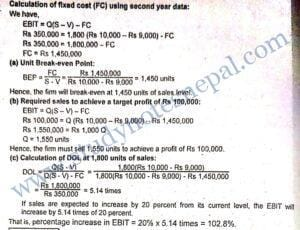4) Given the following information from A and B:

Firm A: Break Even point in units= 25,000; Total fixed cost = Rs. 80,000; Total revenue at BEP = Rs. 200,000

Firm B: Break Even point in units = 30,000; Total fixed cost Rs. 120,000; Total revenue at BEP Rs. 240,000

a. Which firm has the higher operating leverage at any given level of sales? Explain.

b. At what level of sales in units, do both firms earn the same operating profit?

c. If both the companies require an after tax profit of Rs. 36,000; what is the target unit of sales required in each company? Assume the corporate tax rate is 40%.

SOLUTION

a. Other things remaining the same, a firm with larger fixed costs has higher operating leverage at any given level of sales. The fixed cost of firm B is larger so it has higher operating leverage.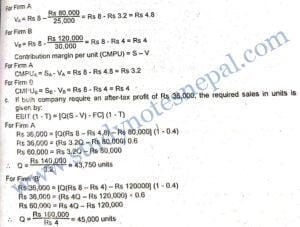5) The following information is given for the purpose of calculating break-even points.

Selling price per unit Rs. 5

Variable cost per unit Rs. 3

Fixed costs (including depreciation of Rs. 8,000) Rs. 24,000

You are required to calculate

(i) operating break-even point,

(ii) cash break-even point,

(iii) operating break-even point if the desired profit is Rs. 30,000 and

(iv) desired profits after income tax is Rs. 20,000 and income tax is 40 percent.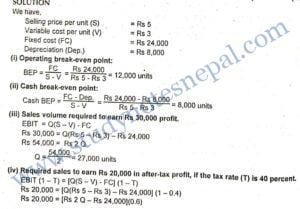Rs 20,000 = Rs 1.2 Q – Rs 14,400

Rs 34,400 = Rs 1.2 Q¹

Q = 34,400 / 1.2 = 28,666.67 units

6) A Toy Manufacturing Company expected to break-even on an accounting basis in its third year. Sales for the third year are projected at 15,000 units. Selling price of a toy is Rs. 30 and variable costs are Rs. 18 each. Installed fixed assets including plant and machinery, furniture and fixtures amounted to Rs. 80,000 which is depreciated on a straight line basis in five years. What will be the fixed costs without depreciation? If fixed costs excluding depreciation are increased by 10 percent, what is its cash break-even point in units? What is the operating break even point in units?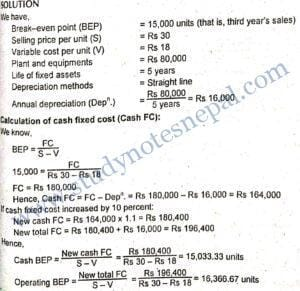7) The following relationships exist for. Detergent Soap Company, a manufacturer of superior quality soaps. Each packet of detergent soap is sold for Rs. 65; the fixed costs are Rs. 180,000, and variable costs are Rs. 35 per packet.

a. What is the company’s gain or loss at sales of 4000 packets?

b. What is the break even volume in rupees?

c. If a company desires to achieve Rs. 50,000 after tax income, what sales volume is required? Assume corporate tax is 20 percent.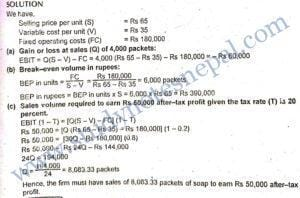###### 1) What is business risk? What are some determinants of business risk?

Business risk is the riskiness on a firm’s stock provided that the firm has used no debt capital. It is the risk inherent in the operation of the business. A firm’s business risk arises because of uncertainty associated with projections of return on invested capital. Return on investment varies due to the number of factors such as variability in demand, price of the product and cost of inputs, general economic condition, competition and so on. Business risk, also called operating risk, is the variation in the return due to the inherent attributes of operation of a firm. The business risk is measured by variability in operating income of the firm. Larger variability in operating income indicates higher business risk. The firm’s business risk changes over time and it varies from firm to firm. Some determinants of business risk are as follows:

(a) Demand volatility: Higher volatility in demand for a firm’s product results in larger variability in its operating income. Thus, a firm with higher demand volatility is exposed to higher business risk.

(b) Selling price volatility: The alternative demand and supply conditions, nature of competitions and many other factors affect the selling price of a firm’s product. Higher the selling price volatility greater will be the fluctuations in operating income leading to higher level of business risk.

(c) Input costs volatility: Cost of inputs changes over the time, which affects the total cost of output. Higher the degree of volatility associated with input costs, higher will be the fluctuations in the firm’s operating cost and hence the operating income. Thus, such firms are exposed to higher levels of business risk.

(d) Efficiency of price adjustment: Change in input costs requires the selling price also to change with a view to maintain stability in operating income. However, the speed of selling price adjustment in response to the change in input costs depends on the firm’s efficiency of price adjustment. Thus, higher price adjustment efficiency of the firm leads to lower level of business risk.

(e) Technological changes: Many firms are exposed to a higher speed of technological changes. Such firms should have higher capacity to adapt to the changing technologies to maintain demand for the firm’s product. Thus, higher the speed of technological changes and lower the capacity of the firm towards technological adaptation, higher will be the level of business risk

(f) Level of fixed operating costs: Firms with higher fixed operating costs must have larger sales to meet the fixed cost. At lower sales levels such firms are not able to meet the fixed operating costs. Thus, such firms are exposed to higher levels of business risk.

###### 2) What is meant by the term ‘leverage’? With which type of risk is each leverage generally associated?

Leverage is a more popular term used in physics, which refers to the use of a lever to raise a heavy object with relatively small forces. In finance leverage refers to the potential use of fixed costs to magnify the earnings. There are three types of leverage: operating leverage, financial leverage and total leverage.

Operating leverage: Operating leverage shows the responsiveness of change in operating profit to the change in sales. A given change in sales usually brings more than proportionate change in operating profit because of the use of fixed operating costs. Thus operating leverage refers to the potential use of fixed cost by a firm. The numerical measure of operating leverage is called the degree of operating leverage (DOL). It measures the extent of change in operating profit as a result of change in sales. It is the ratio of percentage change in operating profit to the percentage change in sales. It is expressed as:

DOL= Percentage change in operating profit /Percentage change in sales

If DOL is 2 times, for example, it implies that a one percent change in sales results in a two percentage change in operating profit. It applies to both directions- an increase in sales results in more than proportionate increase in operating profit and a decrease in sales results in a more than proportionate decrease in operating profits. If all operating costs were variable, there would be nothing like operating leverage. In this case, there would be equal proportionate change in sales and operating profit. Therefore, use of fixed operating costs results in the existence of operating leverage. Other things remaining the same, use of higher fixed operating costs result in higher degree of operating leverage. The degree of operating leverage is associated with the level of operating risk or business risk. In other words, a higher degree of operating leverage implies that the firm has higher business risk. Other things remaining the same, a firm with higher degree of operating leverage should have a higher level of sales than the firm with lower degree of operating leverage to earn the same level of operating profit. Thus, degree of operating leverage has practical implications in assessing the operating or business risk associated with a firm.

Financial leverage: Financial leverage explains how a given change in operating income of a firm affects its earnings per share and earnings to common stockholders. It is the responsiveness of change in firm’s EPS to the change in operating profit. Financial leverage exists because of the use of fixed charge bearing securities, such as bonds and preferred stock. In fact, financial leverage refers to the use of debt in a firm. The numerical measure of financial leverage is called degree of financial leverage (DFL). It shows the extent to which a firm’s earnings per share or earnings to common stockholders change in response to the change in operating profit. Such change is more than proportional. The DFL is worked out using the following relationship:

DFL = Percentage change in EPS/ Percentage change in operating profit

If the degree of financial leverage of a firm, for example, is 1.5 times it implies that a one percentage change in operating profit results in a 1.5 percentage change in earnings per share and earnings to common stockholders in the same direction. A firm with higher fixed financing costs, such as interest and preferred stock dividend. has a higher degree of financial leverage. As the firm employs more and more debt, its interest expenses and return on equity also increase correspondingly. It implies that, in any capital structure, interest expenses and return on equity increase with financial leverage. Higher the financial leverage, more the proportionate change in EPS and ROE for a given level of change in sales and EBIT. The implication of using higher debt is that a firm with higher financial leverage can magnify its ROE with successful use of debt. Debt carries fixed interest and amount earned in excess of fixed interest is available to the common stockholders, hence their return is maximized. However, if the firm is not successful in using debt, interest expense remains as the fixed financial burden. The use of excessive debt increases the financial risk to the common stockholders, because they may feel their position insecure with the increased debt. Thus, financial leverage affects the return and financial risk for stockholders.

Total leverage: Total leverage is the combination of operating and financial leverage. Degree of operating leverage measures the degree of business risk associated with a firm. The operating leverage results from the existence of fixed operating costs. On the other hand, the degree of financial leverage measures the financial risk associated with a firm. It results from the existence of fixed financing costs. The combined use of operating and financial leverage causes considerable change in net income and EPS even if there is only a small change in sales. Such combined effect of operating and financial leverage is numerically measured by degree of total leverage (DTL).

###### 3) What are the factors affecting the target capital structure?

The target capital structure of a firm is affected by various factors as described below:

• Business risk: The level of business risk is determined by the use of fixed operating cost or operating leverage. The chance of business failure is higher for the firm with a higher level of business risk. Thus they tend to use lower debt.
• Cash flow stability: A firm with relatively stable cash flows finds no difficulties in meeting its fixed charge obligation. Thus such firms tend to use more debt.
• Firm size: The larger firms have easy access to the capital market as they have higher credit ratings for debt issues. Therefore they tend to use more debt capital than smaller firms.
• Sales growth: The firms with significant growth in sales have high market price per share. Thus they prefer to use more equity.
• Control and risk: Management’s attitude towards control and risk also affect capital structure decisions. If management wants to maintain control in the firm, they prefer to use more debt financing. Similarly, if the management of a firm is more risk seeker, they attempt to use more debt to take the advantage of financial leverage.
• Debt service capacity: Debt service capacity of the firm is indicated by interest coverage ratio. The firms with higher interest coverage ratio tend to use more debt.
• Asset structure: Maturity structure of assets to be financed also affects the capital structure. A firm with relatively higher longer-term assets and stable demand for products tends to use more long-term debt. On the other hand, a firm with relatively higher investment in receivables and inventory tends to use more short-term funds.